Share

Selina solutions for Class 10 Physics chapter 5 - Refraction through a Lens

Selina ICSE Concise Physics for Class 10

Selina Selina ICSE Concise Physics Class 10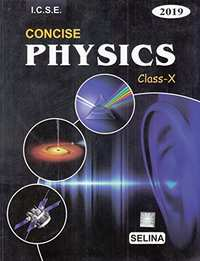Chapter 5: Refraction through a Lens

Chapter 5: Refraction through a Lens solutions [Page 0]

What is a lens?

Name the different kinds of lenses. Draw diagrams to illustrate them.

State two differences between a convex and a concave lens.

Which lens is converging: An equiconcave lens or an equiconvex lens.

Out of the two lenses, one concave and the other convex, state which one will show the divergent action on a light beam.

Show by a diagram the refraction of two light rays incident parallel to the principal axis on a convex lens by treating it as a combination of a glass block and two triangular glass prisms.

Show by a diagram, the refraction of two light rays incident parallel to the principal axis on a concave lens by treating it as a combination of a glass block and two triangular glass prisms.

How does the action of a convex lens differ from that of a concave lens on a parallel beam of light incident on them? Draw diagram to illustrate your answer.

Define the term principal axis of a lens.

Explain optical centre of a lens with the help of proper diagram(s)

A ray of light incident at a point on the principal axis of a convex lens passes undeviated through the lens.
(a) What special name is given to this point on the principal axis?
(b) Draw a labelled diagram to support your answer in part (a)

State the condition when a lens is called an equi-convex or equi-concave

Define the term principal foci of a convex lens and illustrate your answer with the aid of proper diagrams.

Define the term principal foci of a concave lens and show them with the help of proper diagrams.

Draw a diagram to represent the second focus of a concave lens.

Draw a diagram to represent the second focus of a convex lens.

A ray of light, after refraction through a concave lens emerges parallel to the principal axis. (a) draw a ray diagram to show the incident ray and its corresponding emergent ray. (b) The incident ray when produces meets the principal axis at a point. Name the point.

A ray of light after refraction through a convex lens emerges parallel to principal axis. (a) Draw a ray diagram to show it. (b) The incident ray passes through a point on the principal axis. Name the point

A beam of light incident on a convex lens parallel to its principal axis converges at a point on the principal axis. Name the point. Draw a ray diagram to show it.

A beam of light incident on a thin concave lens parallel to its principal axis diverges and appears to come from a point on the principal axis. Name the point. Draw a ray diagram to show it.

Define the term focal length of a lens.

What do you mean by focal plane of a lens?

State the condition for the following a lens has both its focal lengths equal .

State the condition for the following  a ray passes undeviated through the lens .

A parallel oblique beam of light falls on a (i) convex lens, (ii) concave lens. Draw a diagram in each case to show the refraction of light through the lens.

The diagram alongside shows a lens as a combination of a glass block and two prisms.  Name the lens formed by the combination.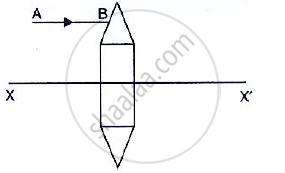The diagram alongside shows a lens as a combination of a glass block and two prisms.

what is the rays the line XX’ called?The diagram alongside shows a lens as a combination of a glass block and two prisms.

Complete the ray diagram and show the path of the incident ray AB after passing through the lensThe diagram alongside shows a lens as a combination of a glass block and two prisms.

The final emergent ray will either meet XX’ at a point or appear to come from a point on XX’. Label the point as F. What is this point called?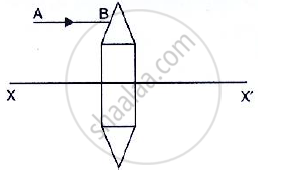The diagram alongside shows a lens as a combination of a glass block and two prisms.
(i) Name the lens formed by the combination.
(ii) what is the line XX’ called?
(iii) Complete the path of the incident ray AB after passing through the lens.
(iv) The final emergent ray either meets XX’ at a point or appears to come from a point on XX’ Label it as F. what is this point called?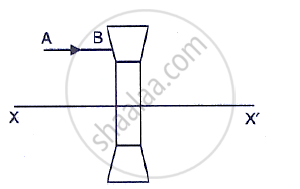In Fig. 5.17, F1 and F2 are the positions of the two foci of the thin lenses shown in diagram (a) and (b) draw accurately the path taken by the light ray AB after it emerges from the lens in each diagram (a) and (b).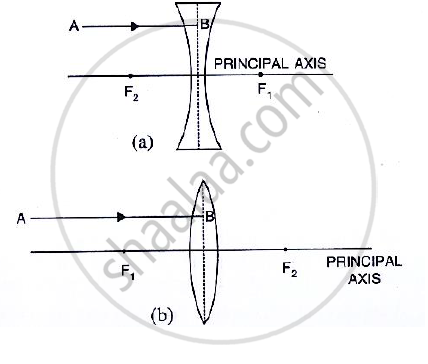In Fig 5.18, F1 and F2 are the two foci of the thin lenses shown in diagram (a) and (b) and AB is the incident ray. Compete the diagram to show the path of the ray AB after refraction through the lens in each diagram (a) and (b).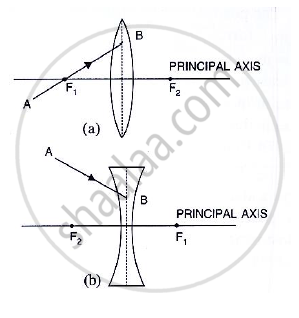Complete the following sentence:
(a) If half part of a convex lens is covered, the focal length ……………… change, but the intensity of image ……………….

Complete the following sentence:

A convex lens is placed in water. Its focal length will ……………………….

Complete the following sentence:

The focal length of a then convex lens is …………….. than that of a thick convex lens.

Chapter 5: Refraction through a Lens solutions [Page 0]

A ray of light after refraction through a lens emerges parallel to the principal axis of the lens. The incident ray either passes through or appears to meet at:
(a) optical centre
(b) first focus
(c) Second focus
(d) centre of curvature of the first surface.

A ray of light incident on a lens parallel to its principal axis, after refraction passes through or appears to come from:
(a) its first focus
(b) its optical centre
(c) its second focus
(d) the centre of curvation of its second surface

Chapter 5: Refraction through a Lens solutions [Page 0]

What are the three principal rays that are drawn to construct the ray diagram for the image formed by a lens? Draw diagrams to support your answer.

In the diagram below, XX’ represents the principal axis, O the optical centre and F the focus of the lens. Complete the path of rays A and B as they emerge out of the lens.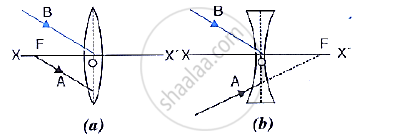Distinguish between a real and a virtual image.

Distinguish between a real and a virtual image.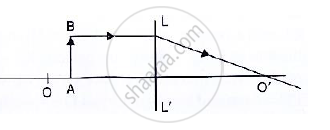Name the lens LL’

Study the diagram (Fig. 5.55) given below.What are the points O and O’ called?

Study the diagram (Fig. 5.55) given below.Complete the diagram to form the image of the object AB.

Study the diagram (Fig. 5.55) given below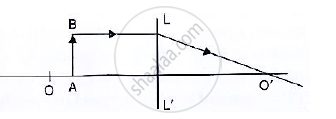state the three characteristics of the image.

Study the diagram (Fig. 5.55) given below.Name a device in which this action of lens is used.

Study the diagram (Fig. 5.54) below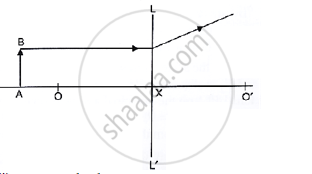Name the lens LL’

LL' is concave lens.what are the points O, O’ called?

Study the diagram (Fig. 5.54) below.Complete the diagram to form the image of the object AB.

Study the diagram (Fig. 5.54) below.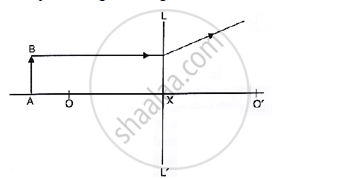State three characteristics of the image

Study the diagram shown in Fig. 5.56

Complete the diagram to show the formation of image A’B’ of the object AB of same size.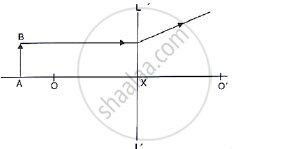Study the diagram shown in Fig. 5.56

Name the lens LL’ and draw its outline.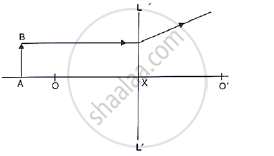Study the diagram shown in Fig. 5.56

what are the points O and O’ called?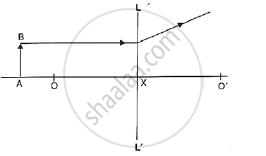Study the diagram shown in Fig. 5.56

where is the object located?Study the diagram shown in Fig. 5.56

Where is the image formed?Study the diagram shown in Fig. 5.56

what are the two other characteristics of the image?The following diagram in Fig. 5.57 shows an object AB and a converging lens L with foci F1 and F2.
(a) Draw two rays from the object and complete the diagram to locate the position of the image. Mark the image CD. Clearly mark on the diagram the position of the eye from where the image can be viewed.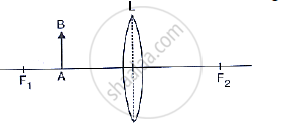The following diagram in Fig. 5.57 shows an object AB and a converging lens L with foci F1 and F2 State three characteristics of the image in relation to the object.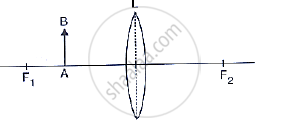The diagram given below in Fig. 5.58 shows the position of an object OA in relation to a converging lens whose foci are at F1 and F2.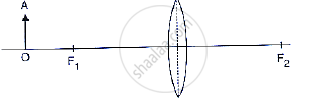draw two rays to locate the position of the image.

The diagram given below in Fig. 5.58 shows the position of an object OA in relation to a converging lens whose foci are at F1 and F2.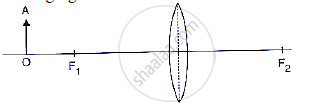State the position of image with reference to the lens.

The diagram given below in Fig. 5.58 shows the position of an object OA in relation to a converging lens whose foci are at F1 and F2.Describe three characteristic of the image.

The diagram given below in Fig. 5.58 shows the position of an object OA in relation to a converging lens whose foci are at F1 and F2.Describe how the distance of the image from the lens and the size of the image change as the object is moved towards F1.

A converging lens forms the image of an object placed in front of it beyond 2F2 of the lens. (a) Where is the object placed?

Draw a ray diagram to show the formation of image.

Where is the image formed?

State three characteristics of the image.

The following diagram in given below shows an object OA and its virtual image IB formed by a lens.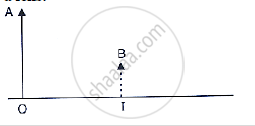The given below figure shows an object OA and its image IB formed by a lens.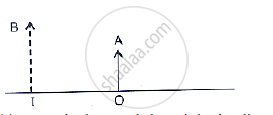The given below figure shows an object OA and its image IB formed by a lens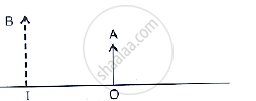draw suitable rays to locate the lens and its focus.

The given below figure shows an object OA and its image IB formed by a lens. State three characteristics of the image.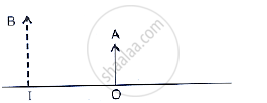A convex lens forms an image of an object equal to the size of the object.Where is the object placed in front of the lens?

A convex lens forms an image of an object equal to the size of the object.draw a diagram to illustrate it.

A convex lens forms an image of an object equal to the size of the object.state two more characteristics of the image.

A lens forms an erect, magnified and virtual image of an object. name the type of lens.

A lens forms an erect, magnified and virtual image of an object.Where is the object placed in relation to the lens?

A lens forms an erect, magnified and virtual image of an object.Draw a ray diagram to show the formation of image.

A lens forms an erect, magnified and virtual image of an object. name the device which uses this principle.

An object is placed on the axis of a lens. An image is formed by refraction in the lens. For all positions of the object on the axis of the lens, the positions of the image are always always between the lens and the object name the lens.

An object is placed on the axis of a lens. An image is formed by refraction in the lens. For all positions of the object on the axis of the lens, the positions of the image are always always between the lens and the object Draw a ray diagram to show it.

An object is placed on the axis of a lens. An image is formed by refraction in the lens. For all positions of the object on the axis of the lens, the positions of the image are always always between the lens and the object.State three characteristics of the image.

Classify as real or virtual, the image of a candle flame formed on a screen by a convex lens. Draw a ray diagram to illustrate how the image is formed.

Show by a ray diagram that a diverging lens cannot form a real image of an object placed anywhere on its principal axis.

Draw a ray diagram to show how a converging lens can form a real and enlarged image of an object.

Where will the image be formed if an object is kept in front of a concave lens at a distance equal to its focal length? Draw a ray diagram to illustrate your answer.

Draw a ray diagram to show how a converging lens is used as a magnifying glass to observe a small object. Mark on your diagram the foci of the lens and the position of the eye.

Draw a ray diagram to show how a converging lens can form an image of the sun. Hence give a reason for the term ‘burning glass’ for a converging lens used in this manner.

A lens forms an inverted image of an object.  what kind of lens is this?

A lens forms an inverted image of an object what is the nature of the image real or virtual?

A lens forms an upright and magnified image of an object  name the lens

A lens forms an upright and magnified image of an object State whether the image is real or virtual

a) name the lens which always forms an erect and virtual image.
(b) state whether the image in part (a) is magnified or diminished.

A lens forms an upright and diminished image of an object irrespective of its position. What kind of lens is this?

Give two characteristic of the image formed by a concave lens.

Give two characteristic of the virtual image formed by a convex lens.

In each of the following cases, where must an object be placed in front of a convex lens so that the image formed is at infinity.

In each of the following cases, where must an object be placed in front of a convex lens so that the image formed is of same size as the object .

In each of the following cases, where must an object be placed in front of a convex lens so that the image formed is inverted and enlarged .

In each of the following cases, where must an object be placed in front of a convex lens so that the image formed is upright and enlarged image?

Complete the following table:

 Type of lens Position of object Nature of image Size of image Convex Between optic centre and focus Convex At focus Concave At infinity Concave At any distance

State the changes in the position, size and nature of the image of an object when brought from infinity up to a convex lens. Illustrate your answer by drawing ray diagrams.

State the changes in the position, size and nature of the image of an object when brought from infinity up to a concave lens. Illustrate your answer by drawing ray diagrams.

Complete the following sentence:

An object is placed at a distance of more than 40 cm from a convex lens of focal length 20 cm. The image formed is real, inverted and ……….

Complete the following sentence:

an object is placed at a distance 2f from a convex lens of focal length f. The size of image formed is ………….. that of the object.

Complete the following sentence:

an object is placed at a distance 5 cm from a convex lens of focal length 10 cm. The image formed is virtual, upright and …………….

State whether the following statement are ‘true’ or ‘false’ by writing T/F against them.

A convex lens has a divergent action and a concave lens has a convergent action.

State whether the following statement are ‘true’ or ‘false’ by writing T/F against them.

A concave lens, if kept at a proper distance from an object, can form its real image.

State whether the following statements\ are ‘true’ or ‘false’ by writing T/F against them.

A ray of light incident parallel to the principal axis of a lens, passes undeviated after refraction.

State whether the following statement are ‘true’ or ‘false’ by writing T/F against them.

A ray of light incident at the optical centre of lens, passes undeviated after refraction

State whether the following statement are ‘true’ or ‘false’ by writing T/F against them.

A concave lens forms a magnified or diminished image depending on the distance of object from it.

Chapter 5: Refraction through a Lens solutions [Page 0]

For an object placed at distance 20 cm in front of a convex lens, the image is at distance 20 cm behind the lens. The focal length of convex lens is:
(a) 20 cm (b) 10 cm (c) 15 cm (d) 40 cm.

For the object placed between optical centre and focus of a convex lens, the image is:
(a) real and enlarged
(b) real and diminished
(c) virtual and enlarged
(d) virtual and diminished

A concave lens forms the image of an object which is:
(a) virtual, inverted and diminished.

(b) virtual, upright and diminished.

(c) virtual, inverted and enlarged.

(d) virtual, upright and enlarged.

Chapter 5: Refraction through a Lens solutions [Page 0]

Define the term power of a lens. In what unit is it expressed?

How is the power of a lens related to its focal length?

How does the power of a lens change is its focal length is doubled?

How is the sign (+ Or -) of power of a lens related to its divergent or convergent action?

The power of a lens is negative. State whether it is convex or concave?

What is magnifying glass? State its two uses.

Draw a neat labelled ray diagram to show the formation of image by a magnifying glass. State three characteristics of the image.

Where is the object placed in reference to the principal focus of a magnifying glass, so as to see its enlarged image? Where is the image obtained?

Define magnifying power of a simple microscope. How can it be increased?

Describe in brief how would you determine the approximate focal length of a convex lens.

The diagram in Fig 5.68 shows the experimental set up for the determination of focal length of a lens using a plane mirror.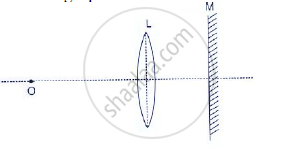(i) draw two rays from the point O of the object to show the formation of image I at O itself.
(ii) What is the size of the image I?
(iii)State two more characteristics of the image I.
(iv) Name the distance of the objects O from the optical centre of the lens.
(v) To what point will the rays return if the mirror is moved away from the lens by a distance equal to the focal length of the lens?

Describe how you would determine the focal length of a converging lens, using a plane mirror and one pin. Draw a ray diagram to illustrate your answer.

State two applications of a convex lens .

State two applications  a concave lens .

How will you differentiate between a convex and a concave lens by looking at (i) a distant object, (ii) a printed page?

Chapter 5: Refraction through a Lens solutions [Page 0]

On reducing the focal length of a lens, its power:
(a) decreases
(b) increases
(c) does not change
(d) first increases then decreases

A magnifying glass forms:
(a) a real and diminished image
(b) a real and magnified image
(c) a virtual and magnified image
(d) a virtual and diminished image

Chapter 5: Refraction through a Lens solutions [Page 0]

The power of a lens is + 2.0 D. Find its focal length and state the kind of the lens.

Express the power (with sign) of a concave lens of focal length 20 cm.

Chapter 5: Refraction through a Lens

Selina Selina ICSE Concise Physics Class 10Selina solutions for Class 10 Physics chapter 5 - Refraction through a Lens

Selina solutions for Class 10 Physics chapter 5 (Refraction through a Lens) include all questions with solution and detail explanation. This will clear students doubts about any question and improve application skills while preparing for board exams. The detailed, step-by-step solutions will help you understand the concepts better and clear your confusions, if any. Shaalaa.com has the CISCE Selina ICSE Concise Physics for Class 10 solutions in a manner that help students grasp basic concepts better and faster.

Further, we at Shaalaa.com are providing such solutions so that students can prepare for written exams. Selina textbook solutions can be a core help for self-study and acts as a perfect self-help guidance for students.

Concepts covered in Class 10 Physics chapter 5 Refraction through a Lens are Image Formation by Lenses - Convex Lens, Image Formation in Lenses Using Ray Diagrams - Concave Lens, Concept of Magnifying Glass, Types of Lenses (Converging and Diverging),, Refraction by a Lens, Power of a Lens, Concept Refraction of Light Through a Lense.

Using Selina Class 10 solutions Refraction through a Lens exercise by students are an easy way to prepare for the exams, as they involve solutions arranged chapter-wise also page wise. The questions involved in Selina Solutions are important questions that can be asked in the final exam. Maximum students of CISCE Class 10 prefer Selina Textbook Solutions to score more in exam.

Get the free view of chapter 5 Refraction through a Lens Class 10 extra questions for Physics and can use Shaalaa.com to keep it handy for your exam preparation

S